### Home > PC > Chapter 8 > Lesson 8.2.4 > Problem8-123

8-123.
1. Find the sum of the following infinite geometric series. If the answer is “∞,” explain why. Homework Help ✎

1.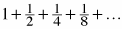2.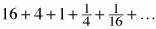3. a + 0.9a + 0.81a + 0.729a + …

4. 1 + 1.1 + 1.21 + 1.331 + …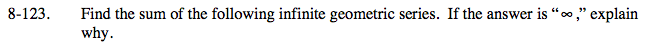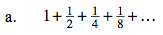$a_n=\left(\frac{1}{2}\right)^{n-1} \ \ \text{Sum}=\frac{1}{1-\frac{1}{2}}=\frac{1}{\frac{1}{2}}=2$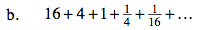$a_n=16\left(\frac{1}{4}\right)^{n-1} \ \ \text{Sum}=\frac{a_1}{1-r}$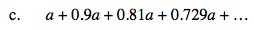$\text{Sum}=\frac{a}{1-r}$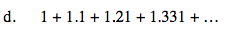The sum would be ∞ because r > 1.